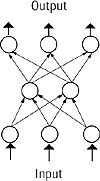# 7.C++ Neural Networks and Fuzzy Logic by Valluru B. Rao M&T Books, IDG Books Worldwide, Inc. ISBN: 1558515526   Pub Date: 06/01/95

## Chapter 1Introduction to Neural Networks

### Neural Processing

How do you recognize a face in a crowd? How does an economist predict the direction of interest rates? Faced with problems like these, the human brain uses a web of interconnected processing elements called neurons to process information. Each neuron is autonomous and independent; it does its work asynchronously, that is, without any synchronization to other events taking place. The two problems posed, namely recognizing a face and forecasting interest rates, have two important characteristics that distinguish them from other problems: First, the problems are complex, that is, you can’t devise a simple step-by-step algorithm or precise formula to give you an answer; and second, the data provided to resolve the problems is equally complex and may be noisy or incomplete. You could have forgotten your glasses when you’re trying to recognize that face. The economist may have at his or her disposal thousands of pieces of data that may or may not be relevant to his or her forecast on the economy and on interest rates.

The vast processing power inherent in biological neural structures has inspired the study of the structure itself for hints on organizing human-made computing structures. Artificial neural networks, the subject of this book, covers the way to organize synthetic neurons to solve the same kind of difficult, complex problems in a similar manner as we think the human brain may. This chapter will give you a sampling of the terms and nomenclature used to talk about neural networks. These terms will be covered in more depth in the chapters to follow.

### Neural Network

A neural network is a computational structure inspired by the study of biological neural processing. There are many different types of neural networks, from relatively simple to very complex, just as there are many theories on how biological neural processing works. We will begin with a discussion of a layered feed-forward type of neural network and branch out to other paradigms later in this chapter and in other chapters.

A layered feed-forward neural network has layers, or subgroups of processing elements. A layer of processing elements makes independent computations on data that it receives and passes the results to another layer. The next layer may in turn make its independent computations and pass on the results to yet another layer. Finally, a subgroup of one or more processing elements determines the output from the network. Each processing element makes its computation based upon a weighted sum of its inputs. The first layer is the input layer and the last the output layer. The layers that are placed between the first and the last layers are the hidden layers. The processing elements are seen as units that are similar to the neurons in a human brain, and hence, they are referred to as cells, neuromimes, or artificial neurons. A threshold function is sometimes used to qualify the output of a neuron in the output layer. Even though our subject matter deals with artificial neurons, we will simply refer to them as neurons. Synapses between neurons are referred to as connections, which are represented by edges of a directed graph in which the nodes are the artificial neurons.

Figure 1.1 is a layered feed-forward neural network. The circular nodes represent neurons. Here there are three layers, an input layer, a hidden layer, and an output layer. The directed graph mentioned shows the connections from nodes from a given layer to other nodes in other layers. Throughout this book you will see many variations on the number and types of layers.Figure 1.1  A typical neural network.

### Output of a Neuron

Basically, the internal activation or raw output of a neuron in a neural network is a weighted sum of its inputs, but a threshold function is also used to determine the final value, or the output. When the output is 1, the neuron is said to fire, and when it is 0, the neuron is considered not to have fired. When a threshold function is used, different results of activations, all in the same interval of values, can cause the same final output value. This situation helps in the sense that, if precise input causes an activation of 9 and noisy input causes an activation of 10, then the output works out the same as if noise is filtered out.

To put the description of a neural network in a simple and familiar setting, let us describe an example about a daytime game show on television, The Price is Right.C++ Neural Networks and Fuzzy Logic
ISBN: 1558515526
EAN: 2147483647
Year: 1995
Pages: 139

Similar book on Amazon